## Example Questions

← Previous 1

### Example Question #1 : How To Find The Slope Of A Line

Refer to the following graph: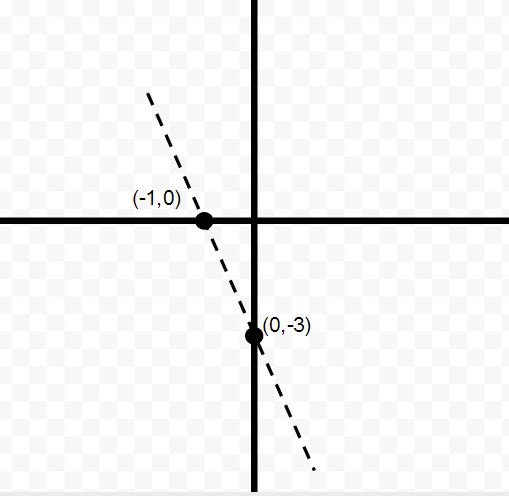What is the slope of the line shown?

1/3

–3

–1/3

3

–1

–3

Explanation:

One can use either the slope formula m = (y2 – y1)/(x2 – x1) or the standard line equation, y = mx + b to solve for the slope, m. By calculation or observation, one can determine that the slope is –3.

### Example Question #2 : How To Find The Slope Of A Line

What is the slope of the equation 4x + 3y = 7?

4/3

–7/3

3/4

–3/4

–4/3

–4/3

Explanation:

We should put this equation in the form of y = mx + b, where m is the slope.

Isolate the y term: 3y = 7 – 4x

Divide by 3: y = 7/3 – 4/3 * x

Rearrange terms: y = –4/3 * x + 7/3, so the slope is –4/3.

### Example Question #3 : How To Find The Slope Of A Line

What is the slope of the equation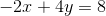?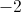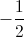Explanation:

To find the slope of a line, you should convert an equation to the slope-intercept form. In this case, the equation would be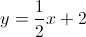, which means the slope is.

### Example Question #4 : How To Find The Slope Of A Line

What is the slope of the line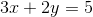?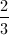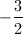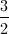Explanation:

To find the slope, put the equation in slope-intercept form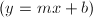. In this case we have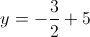, which indicates that the slope is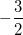.

### Example Question #5 : How To Find The Slope Of A Line

What is the slope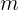of a line passing through the point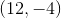, if it is defined by: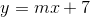?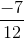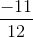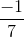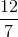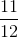Explanation:

Since the equation is defined as it is, you know the y-intercept is.  This is the point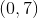.  To find the slope of the line, you merely need to use the two points that you have and find the equation: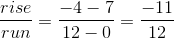### Example Question #1 : How To Find The Slope Of A Line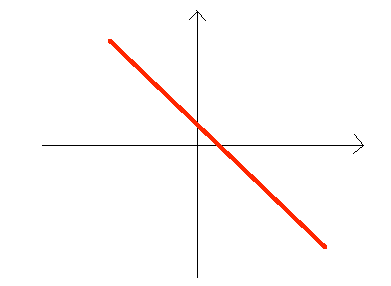Which of the following could be an equation for the red line pictured above?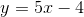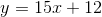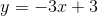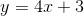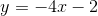Explanation:

There are two key facts to register about this drawing.  First, the line clearly has a negative slope, given that it runs "downhill" when you look at it from left to right.  Secondly, it has a positive y-intercept.  Therefore, you know that the coefficient for theterm must be negative, and the numerical coefficient for the y-intercept must be positive.  This only occurs in the equation.  Therefore, this is the only viable option.

### Example Question #7 : How To Find The Slope Of A Line

What is the slope of a line defined by the equation: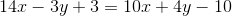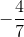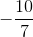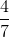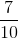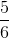Explanation:

A question like this is actually rather easy.  All you need to do is rewrite the equation in slope intercept form, that is:Therefore, begin to simplify:Becomes...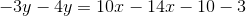Then...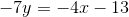Finally, divide both sides by: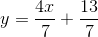The coefficient for theterm is your slope:### Example Question #8 : How To Find The Slope Of A Line

What is the slope of line 3 = 8y - 4x?

2

0.5

-0.5

-2

0.5

Explanation:

Solve equation for y. y=mx+b, where m is the slope

### Example Question #9 : How To Find The Slope Of A Line

Find the slope of the line  6X – 2Y = 14

-6

-3

12

3

3

Explanation:

Put the equation in slope-intercept form:

y = mx + b

-2y = -6x +14

y = 3x – 7

The slope of the line is represented by M; therefore the slope of the line is 3.

### Example Question #10 : How To Find The Slope Of A Line

If 2x – 4y = 10, what is the slope of the line?

2

–2

–5/2

0.5

–0.5

0.5

Explanation:

First put the equation into slope-intercept form, solving for y: 2x – 4y = 10 → –4y = –2x + 10 → y = 1/2*x – 5/2. So the slope is 1/2.

← Previous 1

Tired of practice problems?

Try live online GRE prep today.